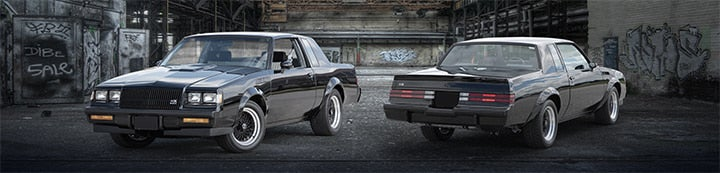1 - 4 of 4 Posts

norbs

·
fantasy tuner/investor
Joined
·
4,035 Posts
Discussion Starter · ·
Here is the pic of the Air filter I want to order for you........Good size? 4" inlet 14" long

Now lets do the math

CFM required for a turbocharged or supercharged motor = Pounds of Boost / 14.7

30/14.7=2.04+1=3 approx

Example: (CID x maximum RPM x #4 ) / 3456

(272 x 6500 x 3)/ 3456

1534 CFM

Sq in required=

To find circumference of a circle multiply diameter by 3.1416

To find diameter of a circle multiply circumference by .31831

CFM Formulas for Filters

Universal Round Taper: (Base + Top) / 2 = A
6+4.625/2=5.3125"

Take A x Length x 6 x 3.14
5.3125 x 14 x 6 x 3.14=1401 CFM

So we are CLOSE!!!!!!!!! for 30 psi boost

turboGUS

·
Registered
Joined
·
703 Posts
air filter

great norbs thanks:cheers:

THE BORG

·
ASSIMILATING HUMANS
Joined
·
98 Posts
great norbs thanks:cheers:
atTEnTion tUrbOgUs. wE cHeCkEd oUr dAtabAnKS and thIs is tHe sAme maTh he useD fOr hIS staGe 1 fLoP. yOu hAvE beEn wArNeD. eNd oF tRaNsmisSiOn.

More_Boost!!

·
Registered
Joined
·
3,328 Posts
The trick here is to KEEP IT CLEAN !!!

They collapse if you don't :yup: (Not me, someone who gave up running filters....)

Here is the pic of the Air filter I want to order for you........Good size? 4" inlet 14" long

Now lets do the math

CFM required for a turbocharged or supercharged motor = Pounds of Boost / 14.7

30/14.7=2.04+1=3 approx

Example: (CID x maximum RPM x #4 ) / 3456

(272 x 6500 x 3)/ 3456

1534 CFM

Sq in required=

To find circumference of a circle multiply diameter by 3.1416

To find diameter of a circle multiply circumference by .31831

CFM Formulas for Filters

Universal Round Taper: (Base + Top) / 2 = A
6+4.625/2=5.3125"

Take A x Length x 6 x 3.14
5.3125 x 14 x 6 x 3.14=1401 CFM

So we are CLOSE!!!!!!!!! for 30 psi boost

1 - 4 of 4 Posts